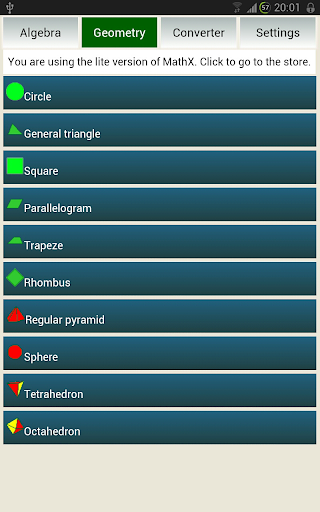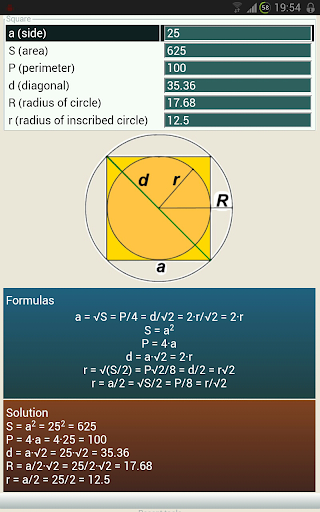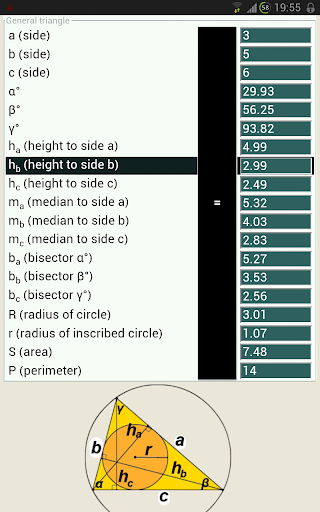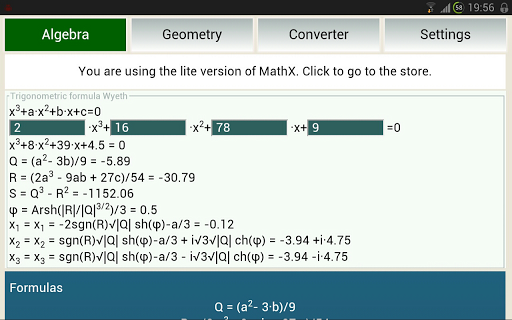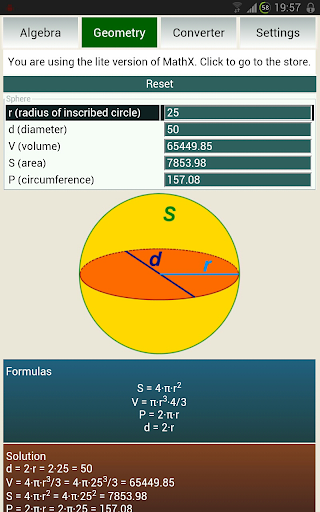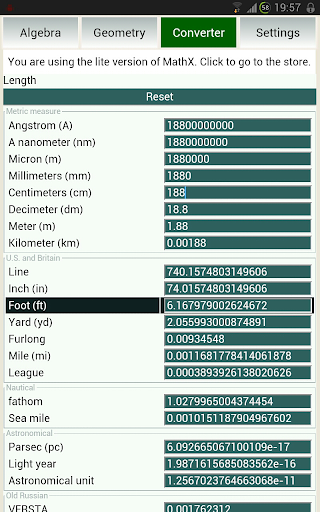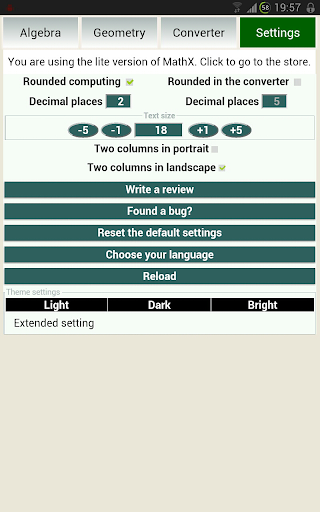MathX allows to calculate most of the parameters of two-dimensional and three-dimensional geometric shapes, convert units of measurement and perform algebraic calculations.

There is one calculator for each figure. It does not matter whether you know the height and the radius or the radius and volume. Just enter the known variables, and the program will choose the formula to find the missing parameters.

No extra clicks. If possible, MathX will immediately write an answer in response on input.
Convenient. For more comfortable use, configure the application to your taste, that suits your needs:
• Rounding of calculations
• The number of decimal places

Effectively. You can swype up and the list of recently-used tools will be available to you.

Informative. You can always see the formula on any tool.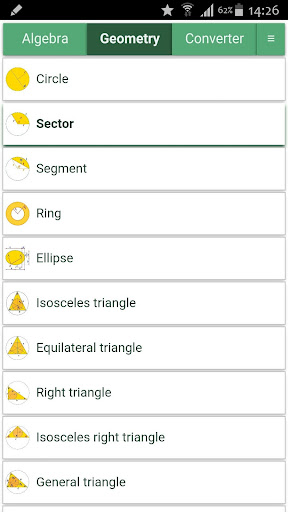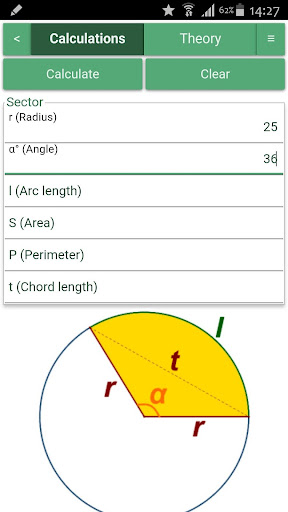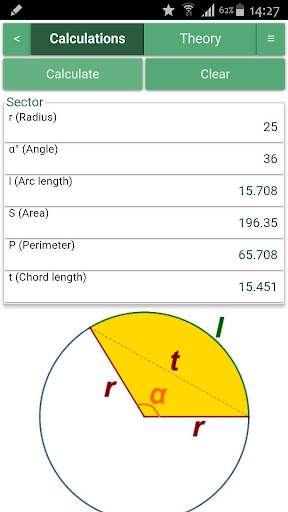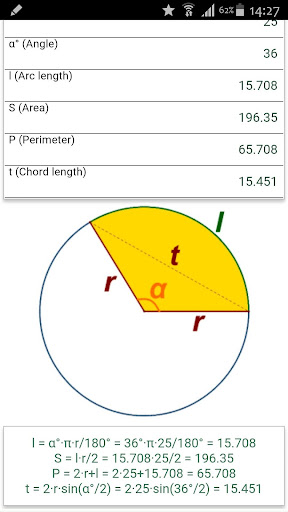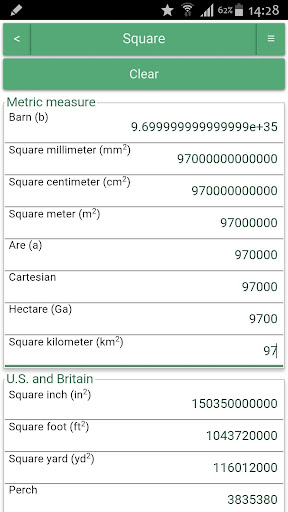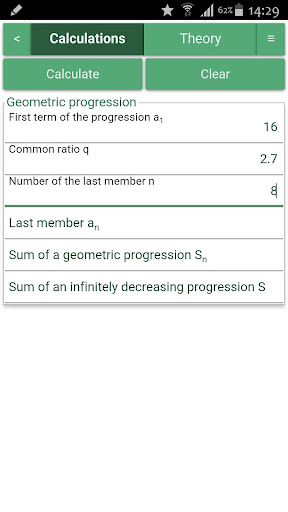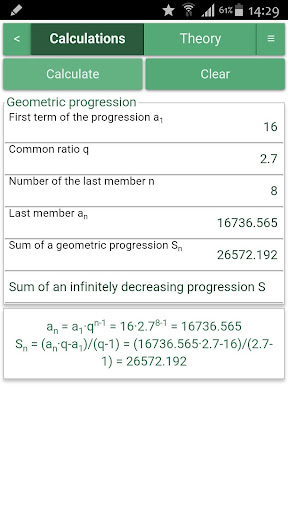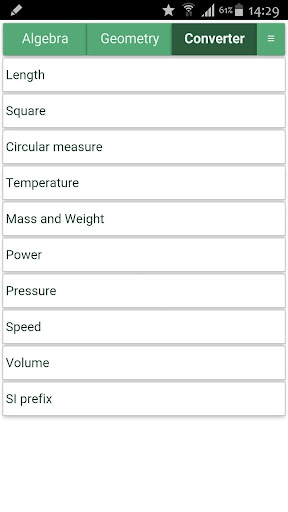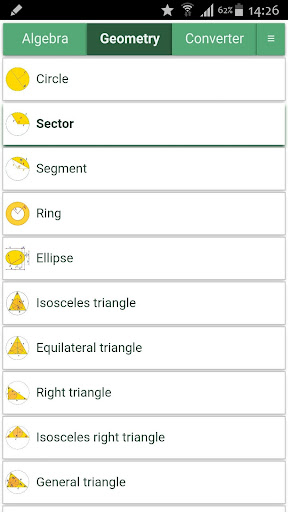Math X is a mathematics program-assistant. It allows to calculate most of the parameters of two-dimensional and three-dimensional geometric shapes, convert units of measurement and perform algebraic calculations.

There is one calculator for each figure. It does not matter whether you know the height and the radius or the radius and volume. Just enter the known variables, and the program will choose the formula to find the missing parameters.

No extra clicks. If possible, MathX will immediately write an answer in response on input.
Convenient. For more comfortable use, configure the application to your taste, that suits your needs:
• Rounding of calculations
• The number of decimal places
• Text Size
• Colours

Effectively. You can swype up and the list of recently-used tools will be available to you.

Informative. You can always see the formula.
1.2.0
• Fixed bugs
• Backbutton
1.1.8
• Solutions available for all figures
• Fixed bugs